# Important Questions CBSE Class 9 Maths Chapter 6-Lines and Angles

CBSE Class 9 Maths Chapter 6 (Lines and Angles) Important Questions with solutions are given here, which can be easily accessed. These questions have been prepared by our experts for students of standard 9 to make them prepare for final exam (2022 – 2023). All the questions are based on CBSE syllabus and taken in reference from NCERT book. Students can do their revision by practising the Important Questions for CBSE Class 9 Maths Chapter 6: Lines and Angles here and score good marks.

To revise the important questions chapter-wise for 9th Maths, reach us at BYJU’S. The chapter lines and angles will consist of topics such as angles formed after the intersection of two lines, linear pair of angles, complementary angles, etc. Let us solve the questions here to understand all the concepts.

Also Check:

## Important Questions & Solutions For Class 9 Chapter 6 (Lines and Angles)

Q.1: In the figure, lines AB and CD intersect at O. If ∠AOC + ∠BOE = 70° and ∠BOD = 40°, find ∠BOE and reflex ∠COE.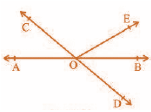Solution:

From the given figure, we can see;

∠AOC, ∠BOE, ∠COE and ∠COE, ∠BOD,  ∠BOE form a straight line each.

So, ∠AOC + ∠BOE +∠COE = ∠COE +∠BOD + ∠BOE = 180°

Now, by substituting the values of ∠AOC + ∠BOE = 70° and ∠BOD = 40° we get:

70° +∠COE = 180°

∠COE = 110°

Similarly,

110° +  40° + ∠BOE = 180°

∠BOE = 30°

Q.2: In the Figure, lines XY and MN intersect at O. If ∠POY = 90° and a : b = 2 : 3, find c.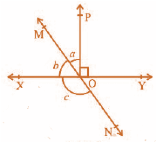Solution:

As we know, the sum of the linear pair is always equal to 180°

So,

∠POY + a + b = 180°

Substituting the value of ∠POY = 90° (as given in the question) we get,

a + b = 90°

Now, it is given that a : b = 2 : 3 so,

Let a be 2x and b be 3x.

∴ 2x + 3x = 90°

Solving this we get

5x = 90°

So, x = 18°

∴ a = 2 × 18° = 36°

Similarly, b can be calculated and the value will be

b = 3 × 18° = 54°

From the diagram, b + c also forms a straight angle so,

b + c = 180°

=> c + 54° = 180°

∴ c = 126°

Q.3: In the Figure, POQ is a line. Ray OR is perpendicular to line PQ. OS is another ray lying between rays OP and OR. Prove that ∠ROS = 1/2(∠QOS – ∠POS).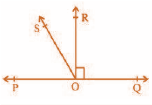Solution:

In the question, it is given that (OR ⊥ PQ) and ∠POQ = 180°

So, ∠POS + ∠ROS + ∠ROQ = 180°    (Linear pair of angles)

Now, ∠POS + ∠ROS = 180° – 90°      (Since ∠POR = ∠ROQ = 90°)

∴ ∠POS + ∠ROS = 90°

Now, ∠QOS = ∠ROQ + ∠ROS

It is given that ∠ROQ = 90°,

∴ ∠QOS = 90° + ∠ROS

Or, ∠QOS – ∠ROS = 90°

As ∠POS + ∠ROS = 90° and ∠QOS – ∠ROS = 90°, we get

∠POS + ∠ROS = ∠QOS – ∠ROS

=>2 ∠ROS + ∠POS = ∠QOS

Or, ∠ROS = ½ (∠QOS – ∠POS) (Hence proved).

Q.4: It is given that ∠XYZ = 64° and XY is produced to point P. Draw a figure from the given information. If ray YQ bisects ∠ZYP, find ∠XYQ and reflex ∠QYP.

Solution: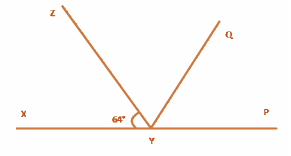Here, XP is a straight line

So, ∠XYZ +∠ZYP = 180°

substituting the value of ∠XYZ = 64° we get,

64° +∠ZYP = 180°

∴ ∠ZYP = 116°

From the diagram, we also know that ∠ZYP = ∠ZYQ + ∠QYP

Now, as YQ bisects ∠ZYP,

∠ZYQ = ∠QYP

Or, ∠ZYP = 2∠ZYQ

∴ ∠ZYQ = ∠QYP = 58°

Again, ∠XYQ = ∠XYZ + ∠ZYQ

By substituting the value of ∠XYZ = 64° and ∠ZYQ = 58° we get.

∠XYQ = 64° + 58°

Or, ∠XYQ = 122°

Now, reflex ∠QYP = 180° + ∠XYQ

We computed that the value of ∠XYQ = 122°. So,

∠QYP = 180° + 122°

∴ ∠QYP = 302°

Q.5: In the Figure, if AB || CD, EF ⊥ CD and ∠GED = 126°, find ∠AGE, ∠GEF and ∠FGE.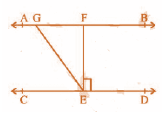Solution:

Since AB || CD GE is a transversal.

It is given that ∠GED = 126°

So, ∠GED = ∠AGE = 126° (alternate interior angles)

Also,

∠GED = ∠GEF + ∠FED

As

EF ⊥ CD, ∠FED = 90°

∴ ∠GED = ∠GEF + 90°

Or, ∠GEF = 126° – 90° = 36°

Again, ∠FGE + ∠GED = 180° (Transversal)

Substituting the value of ∠GED = 126° we get,

∠FGE = 54°

So,

∠AGE = 126°

∠GEF = 36° and

∠FGE = 54°

Q.6: In the Figure, if PQ || ST, ∠PQR = 110° and ∠RST = 130°, find ∠QRS.

[Hint: Draw a line parallel to ST through point R.]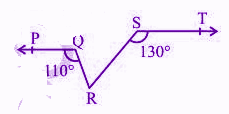Solution:

First, construct a line XY parallel to PQ.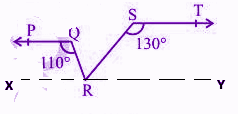As we know, the angles on the same side of the transversal are equal to 180°.

So, ∠PQR + ∠QRX = 180°

Or,∠QRX = 180° – 110°

∴ ∠QRX = 70°

Similarly,

∠RST + ∠SRY = 180°

Or, ∠SRY = 180° – 130°

∴ ∠SRY = 50°

Now, for the linear pairs on the line XY-

∠QRX + ∠QRS + ∠SRY = 180°

Substituting their respective values we get,

∠QRS = 180° – 70° – 50°

Or, ∠QRS = 60°

Q.7: In Fig. 6.33, PQ and RS are two mirrors placed parallel to each other. An incident ray AB strikes the mirror PQ at B, the reflected ray moves along the path BC and strikes the mirror RS at C and again reflects back along CD. Prove that AB || CD.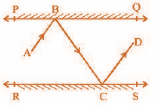Solution:

First, draw two lines BE and CF such that BE  PQ and CF  RS.

Now, since PQ || RS,

So, BE || CF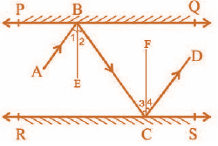BE and CF are normals between the incident ray and reflected ray.

As we know,

Angle of incidence = Angle of reflection (By the law of reflection)

So,

∠1 = ∠2 and

∠3 = ∠4

We also know that alternate interior angles are equal.

Here, BE ⊥ CF and the transversal line BC cuts them at B and C.

So, ∠2 = ∠3 (As they are alternate interior angles)

Now, ∠1 + ∠2 = ∠3 + ∠4

Or, ∠ABC = ∠DCB

So, AB ∥ CD (alternate interior angles are equal)

Q.8: In Fig. 6.40, ∠X = 62°, ∠XYZ = 54°. If YO and ZO are the bisectors of ∠XYZ and ∠XZY respectively of Δ XYZ, find ∠OZY and ∠YOZ.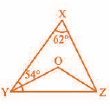Solution:

As we know, the sum of the interior angles of the triangle is 180°.

So, ∠X +∠XYZ + ∠XZY = 180°

substituting the values as given in the question we get,

62° + 54° + ∠XZY = 180°

Or, ∠XZY = 64°

Now, As we know, ZO is the bisector so,

∠OZY = ½ ∠XZY

∴ ∠OZY = 32°

Similarly, YO is a bisector and so,

∠OYZ = ½ ∠XYZ

Or, ∠OYZ = 27° (As ∠XYZ = 54°)

Now, as the sum of the interior angles of the triangle,

∠OZY +∠OYZ + ∠O = 180°

Substituting their respective values we get,

∠O = 180° – 32° – 27°

Or, ∠O = 121°

Q.9: In the figure, if AB || CD || EF, PQ || RS, ∠RQD = 25° and ∠CQP = 60°, then find ∠QRS.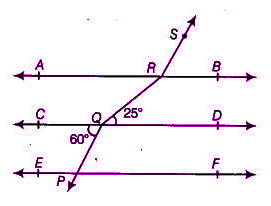Solution:

According to the given figure, we have

AB || CD || EF

PQ || RS

∠RQD = 25°

∠CQP = 60°

PQ || RS.

As we know,

If a transversal intersects two parallel lines, then each pair of alternate exterior angles is equal.

Now, since, PQ || RS

⇒ ∠PQC = ∠BRS

We have ∠PQC = 60°

⇒ ∠BRS = 60° … eq.(i)

We also know that,

If a transversal intersects two parallel lines, then each pair of alternate interior angles is equal.

Now again, since, AB || CD

⇒ ∠DQR = ∠QRA

We have ∠DQR = 25°

⇒ ∠QRA = 25° … eq.(ii)

Using linear pair axiom,

We get,

∠ARS + ∠BRS = 180°

⇒ ∠ARS = 180° – ∠BRS

⇒ ∠ARS = 180° – 60° (From (i), ∠BRS = 60°)

⇒ ∠ARS = 120° … eq.(iii)

Now, ∠QRS = ∠QRA + ∠ARS

From equations (ii) and (iii), we have,

∠QRA = 25° and ∠ARS = 120°

Hence, the above equation can be written as:

∠QRS = 25° + 120°

⇒ ∠QRS = 145°

## Video Lesson on Constructing Angles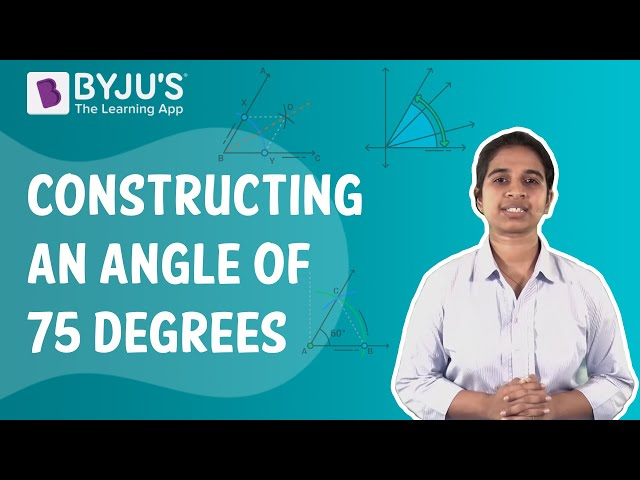### Class 9 Maths Chapter 6 Extra Questions

1. If two lines intersect, prove that the vertically opposite angles are equal.
2. Bisectors of interior ∠B and exterior ∠ACD of a Δ ABC intersect at the point T.Prove that ∠ BTC = ½ ∠ BAC.
3. A transversal intersects two parallel lines. Prove that the bisectors of any pair of corresponding angles so formed are parallel.
4. In the figure, OD is the bisector of ∠AOC, OE is the bisector of ∠BOC and OD ⊥ OE. Show that the points A, O and B are collinear.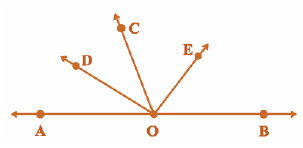5. The angles of a triangle are in the ratio 5 : 3: 7. The triangle is

• An acute-angled triangle
• An obtuse-angled triangle
• A right triangle
• An isosceles triangle

6. Can a triangle have all angles less than 60°? Give a reason for your answer.

7. Can a triangle have two obtuse angles? Give the reason for your answer.

8. How many triangles can be drawn having its angles as 45°, 64° and 72°? Give the reason for your answer.

9. How many triangles can be drawn having its angles as 53°, 64° and 63°? Give the reason for your answer.

10. Two distinct points in the plane determine a _________________ line.

1. doubt in 7 q. how can you prove that if angle 1 and 2 are equal 3 and four are equal?

• they form a ‘z’ that is the shape of alternate interior angles if you look closely so only we conclude it like it

• According to the chapter light angle of incidence is equal to angle of reflection. So angle 1 is incidence and 2 reflected ray same for 3 and 4

• Just like angle 1 and angle 2, angle 3 is incident ray and angle 4 is reflected ray. CF is normal between them. Therefore, by the law of reflection, angle 3 and angle 4 are equal.

• it is based on the law of reflection angle i (incident ray) = angle r (reflected ray).

• both the angles are equal as it was formed by an incident ray and a reflected ray and both the ray measures the same ,so if

• Incident ray is equal ro reflected ray so /_1 + /_2 = /_3 + /_4
another reason is alternate interior angles

• It’s given that the day bisects the angle to form 2 angles. So the two angles are equal. Moreover the incident ray is equal to the reflected ray, so the reflected ray is marked as an incident ray once it comes in contact with the other surface. Hope it helped

2. How do we do the 3rd question??I am having a doubt

3. ∠POS + ∠ROS + ∠ROQ = 180°    (Linear pair of angles)

4. Thanks for the questions.
It was very useful.